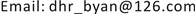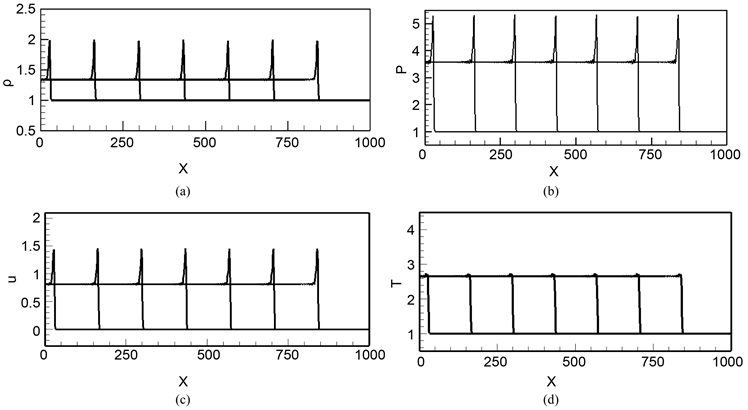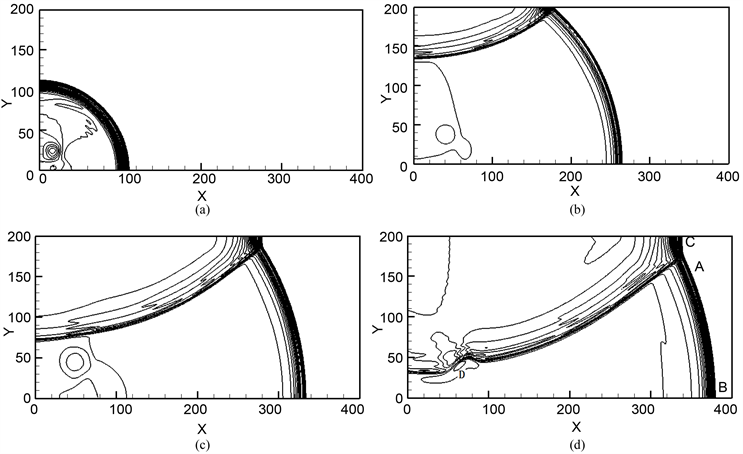﻿ 一种用于双组份爆轰的格子Boltzmann模型 A Lattice Boltzmann Model for Binary Components Detonation

International Journal of Fluid Dynamics
Vol.05 No.04(2017), Article ID:23270,8 pages
10.12677/IJFD.2017.54018

A Lattice Boltzmann Model for Binary Components Detonation

Bo Yan, Jianchao Wang

College of Civil Engineering, Jilin Jianzhu University, Changchun JilinReceived: Dec. 9th, 2017; accepted: Dec. 22nd, 2017; published: Dec. 29th, 2017ABSTRACT

In this paper, we present a lattice Boltzmann model for simulating the binary components detonation phenomena. For modeling the flow behavior in the detonation process, we employ two distribution functions for the density, momentum and energy of reactant and product, respectively. The Lee-Tarver model is selected to describe the chemical reaction kinetics. The reaction heat is naturally coupled with the ﬂow behavior. The numerical examples show that the scheme can be used to simulate the detonation phenomena.

Keywords:Lattice Boltzmann Model, Binary Components, Detonation Phenomena1. 引言

2. 格子Boltzmann模型

2.1. 描述流动的双组份格子Boltzmann模型

$\frac{\partial {f}_{ki}^{\sigma }}{\partial t}+{v}_{ki}\cdot \frac{\partial {f}_{ki}^{\sigma }}{\partial r}=-\frac{1}{{\tau }^{\sigma }}\left[{f}_{ki}^{\sigma }-{f}_{ki}^{\sigma ,eq}\right]$ . (1)

${\rho }^{\sigma }={m}^{\sigma }\sum _{ki}{f}_{ki}^{\sigma }$ . (2)

${n}^{\sigma }{u}^{\sigma }=\sum _{ki}{f}_{ki}^{\sigma }{v}_{ki}$ , (3)

$u=\frac{{\rho }^{r}{u}^{r}+{\rho }^{p}{u}^{p}}{{\rho }^{r}+{\rho }^{p}}$ . (4)

${\rho }^{\sigma }{T}^{\sigma }={P}^{\sigma }={e}_{therm}^{\sigma }={m}^{\sigma }\sum _{ki}\frac{1}{2}{v}_{vi}^{2}{f}_{ki}^{\sigma }-\frac{1}{2}{\rho }^{2}{\left({u}^{\sigma }\right)}^{2}$ , (5)

$T=\frac{{\rho }^{r}{T}^{r}+{\rho }^{p}{T}^{p}}{{\rho }^{r}+{\rho }^{p}}$ . (6)

$\sigma$ 组份的局域平衡态分布函数 ${f}_{ki}^{\sigma ,eq}$ 由下式给出

$\begin{array}{c}{f}_{ki}^{\sigma ,eq}={n}^{\sigma }{F}_{k}\left\{\left[1-\frac{{u}^{2}}{2{\theta }^{\sigma }}+\frac{{u}^{4}}{8{\left({\theta }^{\sigma }\right)}^{2}}\right]+\frac{{v}_{ki\epsilon }{u}_{\epsilon }}{{\theta }^{\sigma }}\left(1-\frac{{u}^{2}}{2{\theta }^{\sigma }}\right)+\frac{{v}_{ki\epsilon }{v}_{ki\pi }{u}_{\epsilon }{u}_{\pi }}{2{\left({\theta }^{\sigma }\right)}^{2}}\left(1-\frac{{u}^{2}}{2{\theta }^{\sigma }}\right)\\ \text{\hspace{0.17em}}\text{\hspace{0.17em}}+\frac{{v}_{ki\epsilon }{v}_{ki\pi }{v}_{ki\upsilon }{u}_{\epsilon }{u}_{\pi }{u}_{\upsilon }}{6{\left({\theta }^{\sigma }\right)}^{3}}+\frac{{v}_{ki\epsilon }{v}_{ki\pi }{v}_{ki\upsilon }{v}_{ki\xi }{u}_{\epsilon }{u}_{\pi }{u}_{\upsilon }{u}_{\xi }}{24{\left({\theta }^{\sigma }\right)}^{4}}\right\}\end{array}$ , (7)

${m}^{\sigma }\sum _{ki}{v}_{ki\alpha }{v}_{ki\beta }{f}_{ki}^{\sigma ,eq}={e}_{therm}^{\sigma }{\delta }_{\alpha \beta }+{\rho }^{\sigma }{u}_{\alpha }^{\sigma }{u}_{\beta }^{\sigma }$ , (8)

${m}^{\sigma }\sum _{ki}{v}_{ki\alpha }{v}_{ki\beta }{v}_{ki\gamma }{f}_{ki}^{\sigma ,eq}={e}_{therm}^{\sigma }\left({u}_{\gamma }^{\sigma }{\delta }_{\alpha \beta }+{u}_{\alpha }^{\sigma }{\delta }_{\beta \gamma }+{u}_{\beta }^{\sigma }{\delta }_{\gamma \alpha }\right)+{\rho }^{\sigma }{u}_{\alpha }^{\sigma }{u}_{\beta }^{\sigma }{u}_{\gamma }^{\sigma }$ , (9)

${m}^{\sigma }\sum _{ki}\frac{1}{2}{v}_{k}^{2}{v}_{ki\alpha }{f}_{ki}^{\sigma ,eq}=2{e}_{therm}^{\sigma }{u}_{\alpha }^{\sigma }+\frac{1}{2}{\rho }^{\sigma }{\left({u}^{\sigma }\right)}^{2}{u}_{\alpha }^{\sigma }$ , (10)

${m}^{\sigma }\sum _{ki}\frac{1}{2}{v}_{k}^{2}{v}_{ki\alpha }{v}_{ki\beta }{f}_{ki}^{\sigma ,eq}=\left[2\frac{{T}^{\sigma }}{{m}^{\sigma }}+\frac{1}{2}{\left({u}^{\sigma }\right)}^{2}\right]{e}_{therm}^{\sigma }{\delta }_{\alpha \beta }+\left[3{e}_{therm}^{\sigma }+\frac{1}{2}{\rho }^{\sigma }{\left({u}^{\sigma }\right)}^{2}\right]{u}_{\alpha }^{\sigma }{u}_{\beta }^{\sigma }$ , (11)

$\begin{array}{c}{f}_{kiI}^{\sigma ,new}={f}_{kiI}^{\sigma }-\frac{{c}_{ki\alpha }}{2}\left({f}_{kiI+1}^{\sigma }-{f}_{kiI-1}^{\sigma }\right)-\frac{\Delta t}{\tau }\left({f}_{kiI}^{\sigma }-{f}_{kiI}^{\sigma ,eq}\right)+\frac{{c}_{ki\alpha }^{2}}{2}\left({f}_{kiI+1}^{\sigma }-2{f}_{kiI}^{\sigma }+{f}_{kiI-1}^{\sigma }\right)\\ \text{\hspace{0.17em}}\text{\hspace{0.17em}}+\frac{{c}_{ki\alpha }\left(1-{c}_{ki\alpha }^{2}\right)}{12}\left({f}_{kiI+2}^{\sigma }-2{f}_{kiI+1}^{\sigma }+2{f}_{kiI-1}^{\sigma }-{f}_{kiI-2}^{\sigma }\right)+\frac{{\theta }_{\alpha I}^{\sigma }|{k}_{\alpha }^{\sigma }|\left(1-|{k}_{\alpha }^{\sigma }|\right)}{2}\left({f}_{kiI+1}^{\sigma }-2{f}_{kiI}^{\sigma }+{f}_{kiI-1}^{\sigma }\right).\end{array}$ (12)

2.2. Lee-Tarver反应率函数

$\frac{\text{d}\lambda }{\text{d}t}=\left\{\begin{array}{l}a\left(1-\lambda \right)+b\left(1-\lambda \right)\lambda ,\text{\hspace{0.17em}}\text{\hspace{0.17em}}T\ge {T}_{th},且0\le \lambda \le 1\\ 0,\text{\hspace{0.17em}}\text{\hspace{0.17em}}\text{\hspace{0.17em}}其它\end{array}$ (13)

$\frac{\partial \lambda }{\partial t}+u\nabla \lambda =0$ ,(14)

$\frac{{\lambda }_{I}^{n+1}-{\lambda }_{I}^{n}}{\Delta t}=-\left\{\begin{array}{l}\frac{u\left({\lambda }_{I}^{n}-{\lambda }_{I-1}^{n}\right)}{\Delta x},u\ge 0\\ \frac{u\left({\lambda }_{I+1}^{n}-{\lambda }_{I}^{n}\right)}{\Delta x},u<0\end{array}$ ,(15)

$\frac{\partial \lambda }{\partial t}=a\left(1-\lambda \right)+b\left(1-\lambda \right)\lambda$ , (16)

${\lambda }_{I}^{n+1}=\frac{{\text{e}}^{\left(a+b\right)\Delta t}+a\left({\lambda }_{I}^{n}-1\right)/\left(a+b{\lambda }_{I}^{n}\right)}{{\text{e}}^{\left(a+b\right)\Delta t}+b\left(1-{\lambda }_{I}^{n}\right)/\left(a+b{\lambda }_{I}^{n}\right)}$ .(17)

2.3. 化学反应与流动的耦合

$\stackrel{˙}{e}={\stackrel{˙}{e}}_{therm}+{\stackrel{˙}{e}}_{chem}$ , (18)

${\stackrel{˙}{e}}_{chem}=\stackrel{˙}{\lambda }\rho Q$ .(19)

${\rho }^{r,new}={\rho }^{r}-\stackrel{˙}{\lambda }\rho$ ,(20)

${\rho }^{p,new}={\rho }^{p}+\stackrel{˙}{\lambda }\rho$ . (21)

3. 数值例子

3.1. 带有黏性和热传导的活塞问题

${\left(\rho ,u,v,T,\lambda \right)}_{L}=\left(1.35,0.81,0,2.65,1\right)$ , (22)

${\left(\rho ,u,v,T,\lambda \right)}_{R}=\left(1,0,0,1,0\right)$ . (23)Figure 1. Physical quantity profiles for the piston problem including effects of viscosity and heat conduction at times 0, 0.05, 0.1, 0.15, 0.2, 0.25 and 0.3: (a) density $\rho$ ; (b) pressure $P$ ; (c) x-component of velocity $u$ ; (d) temperature $T$

${\rho }_{0}\left(D-{u}_{0}\right)={\rho }_{1}\left(D-{u}_{1}\right)$ , (24)

${P}_{1}-{P}_{0}={\rho }_{0}\left(D-{u}_{0}\right)\left({u}_{1}-{u}_{0}\right)$ , (25)

${e}_{1}-{e}_{0}=0.5\left({P}_{1}+{P}_{0}\right)\left(1/{\rho }_{0}-1/{\rho }_{1}\right)+\lambda Q$ , (26)

3.2. 爆轰波的正规反射和Mach反射

$\left(\rho ,u,v,T,\lambda \right)=\left(1.36,0.82,0,2.60,1\right)$ ，在A点和B点处，

$\left(\rho ,u,v,T,\lambda \right)=\left(1,0,0,1,0\right)$ ，其他位置.Figure 2. Sketch of regular and Mach reflections of detonation waveFigure 3. The transition process from regular reflection to Mach reflection: (a) Ignition arises and detonation waves generate; (b) The regular reflection of detonation wave; (c) Conversion from the regular reflection to Mach reflection; (d) The Mach reflection of detonation wave

4. 结论

A Lattice Boltzmann Model for Binary Components Detonation[J]. 流体动力学, 2017, 05(04): 161-168. http://dx.doi.org/10.12677/IJFD.2017.54018

1. 1. Watari, M. and Tsu-tahara, M. (2003) Two Dimensional Thermal Model of the Finite-Difference Lattice Boltzmann Method with High Spatial Isotropy. Physical Review E, 67, Article ID: 036306. https://doi.org/10.1103/PhysRevE.67.036306

2. 2. Gan, Y.B., Xu, A.G., Zhang, G.C., Yu, X.J. and Li, Y.J. (2008) Two-Dimensional Lattice Boltzmann Model for Compressible Flows with High Mach Number. Physica A, 387, 1721-1732. https://doi.org/10.1016/j.physa.2007.11.013

3. 3. Courant, R. and Friedrichs, K. (1948) Supersonic Flow and Shock Waves. In-terscience Publishers Inc., New York.

4. 4. Karni, S. (1994) Multicomponent Flow Calculations by a Consistent Primitive Algorithm. Journal of Computational Physics, 112, 31-43. https://doi.org/10.1006/jcph.1994.1080

5. 5. Shyue, K. (1998) An Efficient Shock-Capturing Algorithm for Compressible Multicomponent Problems. Journal of Computational Physics, 142, 208-242. https://doi.org/10.1006/jcph.1998.5930

6. 6. Marquina, A. and Mulet, P. (2003) A Flux-split Algorithm Applied to Conservative Models for Multicomponent Compressible Flows. Journal of Computational Physics, 185, 120-138. https://doi.org/10.1016/S0021-9991(02)00050-5

7. 7. Loubre, R., Maire, P., Shashkov, M., Breil, J. and Galera, S. (2010) ReALE: A Reconnection-Based Arbitrary-Lagrangian-Eulerian Method. Journal of Computational Physics, 229, 4724-4761. https://doi.org/10.1016/j.jcp.2010.03.011

8. 8. 楼建锋, 于恒. CTVD格式数值计算非均质炸药爆轰问题[J]. 计算物理, 2005, 22(4): 358-364.

9. 9. Galera, S., Maire, P. and Breil, J. (2010) A Two-Dimensional Unstructured Cell-Centered Multi-Material ALE Scheme Using VOF Interface Reconstruction. Journal of Computational Physics, 229, 5755-5787. https://doi.org/10.1016/j.jcp.2010.04.019

10. 10. Osher, S. and Fedkiw, R. (2001) Level Set Methods: An Overview and Some Recent Results. Journal of Computational Physics, 169, 463-502. https://doi.org/10.1006/jcph.2000.6636

11. 11. Sussman, M., Smereka, P. and Osher, S. (1994) A Level Set Approach for Computing Solutions to Incompressible Two-phase Flow. Journal of Computational Physics, 114, 146-159. https://doi.org/10.1006/jcph.1994.1155

12. 12. Scardovelli, R. and Zaleski, S. (2003) Direct Numerical Simulation of Free-Surface and Interfacial Flow. Annual Review of Fluid Mechanics, 31, 567-603. https://doi.org/10.1146/annurev.fluid.31.1.567

13. 13. Tryggvason, G., Bunner, B., Esmaeeli, A., Juric, D., Al-Rawahi, N., Tauber, W., Han, J., Nas, S., and Jan, Y.J. (2001) A Front-Tracking Method for the Computations of Multiphase Flow. Journal of Computa-tional Physics, 169, 708-759. https://doi.org/10.1006/jcph.2001.6726

14. 14. Mao, D. (2007) Towards Front-Tracking Based on Conservation in Two Space Di-mensions II, Tracking Discontinuities in Capturing Fashion. Journal of Computational Physics, 222, 1550-1588. https://doi.org/10.1016/j.jcp.2007.06.004

15. 15. Filippova, O. and Hanel, D. (2000) A Novel Lattice BGK Approach for Low Mach Number Combustion. Journal of Computational Physics, 158, 139-160. https://doi.org/10.1006/jcph.1999.6405

16. 16. Filippova, O. and Hanel, D. (2000) A Novel Numerical Scheme for Reactive Flows at Low Mach Numbers. Computer Physics Communications, 129, 267-274. https://doi.org/10.1016/S0010-4655(00)00113-2

17. 17. Succi, S., Bella, G. and Papetti, F. (1997) Lattice Kinetic Theory for Numerical Combustion. Journal of Scientific Computing, 12(4), 395-408.

18. 18. Yamamoto, K., He, X. and Doolen, G. (2002) Simulation of Combustion Field with Lattice Boltzmann Method. Journal of Statistical Physics, 107, 367-383. https://doi.org/10.1023/A:1014583226083

19. 19. Yan, B., Xu, A.G., Zhang, G.C., Ying, Y.J. and Li, H. (2013) Lattice Boltzmann Model for Combustion and Detonation. Frontiers of Physics, 8, 94-110. https://doi.org/10.1007/s11467-013-0286-z

20. 20. Lin, C.D., Xu, A.G., Zhang, G.C., LI, Y.J. and Succi, S. (2014) Polar-Coordinate Lattice Boltzmann Modeling of Compressible Flows. Physical Review E Statistical Nonlinear & Soft Matter Physics, 89, Article ID: 013307. https://doi.org/10.1103/PhysRevE.89.013307

21. 21. Xu, A.G., Lin, C.D., Zhang, G.C. and Li, Y.J. (2015) Multi-ple-Relaxation-Time Lattice Boltzmann Kinetic Model for Combustion. Physical Review E Statistical Nonlinear & Soft Matter Physics, 91, Article ID: 043306. https://doi.org/10.1103/PhysRevE.91.043306

22. 22. Lee, E.L. and Tarver, C.M. (1980) Phenomenological Model of Shock Initia-tion in Heterogeneous Explosives. Physics of Fluids, 23, 2362-2372. https://doi.org/10.1063/1.862940